# Transistor Base voltage and Current

#### pinkyponky

Joined Nov 28, 2019
338
Hello All!

1) How much base voltage and current needs to be applied to draw maximum collector current of 500mA using 'PDTD114EU'?.

2) The half of the voltage will be present after R1 resistor. Right?. If I applied 5V before R1, then after R1 resistor the voltage will be 2.5V, which will applied to the base of the transistor, Right?.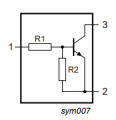The datasheet is attached below.
https://assets.nexperia.com/documents/data-sheet/PDTD1XXXU_SER.pdf

#### AnalogKid

Joined Aug 1, 2013
10,776
2. Not really. The base-emitter junction clamps the voltage across R2 to Vbe. This increases the current through R1, increasing the voltage across R1. Even if R1 = R2, the voltage at the R1-R2 node is not Vin / 2.

1. According to the datasheet, the transistor gain is somewhere between 200 and 400 depending on the ambient air temperature around the part. The datasheet does not have a chart for Vbe versus Ib. If you assume a typical value of 0.6 V, Then using Ohm's Law twice you can calculate the voltage at pin 1 needed for the correct current through the base-emitter junction.

ak

https://assets.nexperia.com/documents/data-sheet/PDTD1XXXU_SER.pdf

#### crutschow

Joined Mar 14, 2008
33,325
1) How much base voltage and current needs to be applied to draw maximum collector current of 500mA using 'PDTD114EU'?.
Are you using the transistor as a switch?
If so, you cannot get enough current through that transistor's 10kΩ input resistor to turn-on the transistor into saturation, as it would require about 250V input voltage, which would burn up the transistor.
2) The half of the voltage will be present after R1 resistor. Right?. If I applied 5V before R1, then after R1 resistor the voltage will be 2.5V, which will applied to the base of the transistor, Right?.
Nope.
You need to do some more study of how BJT's work.
The base to emitter of an NPN transistor looks like a forward-biased diode, which is a low impedance and is below a volt for normal values of base current.

#### WBahn

Joined Mar 31, 2012
29,483
@pinkyponky This looks like homework of some kind. Is it?

#### pinkyponky

Joined Nov 28, 2019
338

#### WBahn

Joined Mar 31, 2012
29,483
Hello All!

1) How much base voltage and current needs to be applied to draw maximum collector current of 500mA using 'PDTD114EU'?.

2) The half of the voltage will be present after R1 resistor. Right?. If I applied 5V before R1, then after R1 resistor the voltage will be 2.5V, which will applied to the base of the transistor, Right?.

View attachment 301100

The datasheet is attached below.
https://assets.nexperia.com/documents/data-sheet/PDTD1XXXU_SER.pdf
500 mA is the absolute max current rating -- you should never intentionally run parts at their absolute max ratings (or even particularly close to them).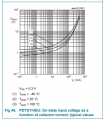At an ambient temperature of 25°C, this shows that you need 40 V to 50 V between pins 1 and 2 to get 500 mA though the collector at a 300 mV Vce.

Even with a mounting that has a 425 mW max power dissipation, at 500 mA that only lets you have a Vce of 0.85 V.

#### pinkyponky

Joined Nov 28, 2019
338
Then, Is that the same voltage as applied voltage after R1?. Could you explain?

#### WBahn

Joined Mar 31, 2012
29,483
Then, Is that the same voltage as applied voltage after R1?. Could you explain?
Not all of the current that goes into R1 goes through R2, some of it goes into the base of the transistor.

The data sheet provides a chart specifically to tell you how much voltage you typically need to get 500 mA through the transistor and keep it somewhat is saturation -- it's about 50 V (which also happens to be, not coincidentally, the maximum input voltage specified in the data sheet in Table 6).

#### pinkyponky

Joined Nov 28, 2019
338
Hello WBahn!

If I applied 10V to R1 resistor then, what voltage can I measured after R1?. Is it same as 10V? or 5V or somthing else?.

#### crutschow

Joined Mar 14, 2008
33,325
Is that the same voltage as applied voltage after R1?. Could you explain?
If you read my post, I did explain.
I said the base-emitter looks like a forward-biased diode and the voltage is less that 1V, so that, of course is the voltage across R2.
What about that don't you understand?

#### pinkyponky

Joined Nov 28, 2019
338
If you read my post, I did explain.
I said the base-emitter looks like a forward-biased diode and the voltage is less that 1V, so that, of course is the voltage across R2.
What about that don't you understand?
So, the voltage at after R1 (transistor base) will be:
5V-0,7V (Diode drop)=4,3V (if 5V applied to R1)
10V-0,7V (Diode drop)=9,3V (if 10V applied to R1)

Am I correct?.

Last edited:

#### WBahn

Joined Mar 31, 2012
29,483
Hello WBahn!

If I applied 10V to R1 resistor then, what voltage can I measured after R1?. Is it same as 10V? or 5V or somthing else?.
The voltage across R2 is the exact same voltage as the voltage between the base and the emitter of the transistor, Vbe.

Under normal conditions, this voltage is clamped, by the base-emitter PN junction, to be about 600 mV to 800 mV for typical silicon transistors.

It's actually pretty easy to determine what the voltage actually is if you have one of the transistors available.

Apply 10 V between pins 1 and 2 and measure the total current draw. This current is flowing through R1, which is nominally 10 kΩ. Let's say that it turns out to be 0.94 mA. That means that there is 9.4 V dropped across R1, leaving 600 mV across R2. That, then, means that there is 60 µA of current flowing in R2 and that the remaining 880 µA is flowing into the base of the transistor.

But keep in mind that the base resistor can vary from the nominal value by up to 30% and the base-emitter resistor can differ from the base resistor by as much as 10%.

#### pinkyponky

Joined Nov 28, 2019
338
Apply 10 V between pins 1 and 2 and measure the total current draw. This current is flowing through R1, which is nominally 10 kΩ. Let's say that it turns out to be 0.94 mA. That means that there is 9.4 V dropped across R1, leaving 600 mV across R2. That, then, means that there is 60 µA of current flowing in R2 and that the remaining 880 µA is flowing into the base of the transistor.
Why will there be a 9.4V voltage drop across R1?. The diode drop will be 0.6V to 0.8V only, right?. So, from my understanding, 9.4V should be across R2, and there will be 0.7V drop across the diode.
What I wrote on post #12 is wrong?.

Last edited:

#### Bordodynov

Joined May 20, 2015
3,116
•pinkyponky

#### crutschow

Joined Mar 14, 2008
33,325
So, the voltage at after R1 (transistor base) will be:
5V-0,7V (Diode drop)=4,3V (if 5V applied to R1)
10V-0,7V (Diode drop)=9,3V (if 10V applied to R1)

Am I correct?.
It would appear you are not.
The voltage from the base to ground across R2 will be 0.7V (approx.).
Note that the BE junction is in parallel with R2 to ground.

Perhaps the simulation below will help:
It shows the input voltage (green trace) versus the base voltage (yellow trace) and the voltage across R2 (Vin-Vbase , red trace).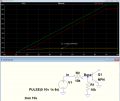Last edited:
•pinkyponky

#### BobTPH

Joined Jun 5, 2013
8,069
You need to go back and read the first chapter of any introductory electronics book. You do not understand parallel and series circuits, let alone voltage dividers.

#### pinkyponky

Joined Nov 28, 2019
338
Hi Crutschow!

Thank you for nice explanation.

I have a plan to use this transistor to drive the 16-Segment display. I have some questions in the below datasheet is attached.

https://www.kingbrightusa.com/images/catalog/SPEC/PSA23-11SURKWA.pdf

The below screenshot is taken from the page 2 of the above datasheet.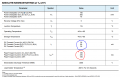Let's discuss the 60mA current (marked in Blue). My question here is, is that current consumption of 60mA is for all segments that are listed on the lift side of the above table, such as B,C,E,F,G,H,J,L,M,N; (or) only for one segment?.

My 2nd question is concerned about the Peak Forward Current of 370mA. So, I need to have a transistor that can stand with and can drive the collector current of 370mA, when the peak forward current occurs.

Could you help me with this?.

Thank you!

#### crutschow

Joined Mar 14, 2008
33,325
Let's discuss the 60mA current (marked in Blue). My question here is, is that current consumption of 60mA is for all segments that are listed on the lift side of the above table, such as B,C,E,F,G,H,J,L,M,N; (or) only for one segment?.

My 2nd question is concerned about the Peak Forward Current of 370mA. So, I need to have a transistor that can stand with and can drive the collector current of 370mA, when the peak forward current occurs.
Those are plainly stated as the ABSOLUTE MAXIMUM RATINGS, which means you should normally operate well below those values.
What about that chart title don't you understand?

Below are typical values you should be using: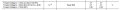#### pinkyponky

Joined Nov 28, 2019
338
Those are plainly stated as the ABSOLUTE MAXIMUM RATINGS, which means you should normally operate well below those values.
What about that chart title don't you understand?

Below are typical values you should be using:
View attachment 301196
Hi Again!

Is that 20mA current consumption is for an one segment (Or) for all segments such as B,C,E,F,G,H,J,L,M,N?.